MCAT Social and Behavioral Sciences : Other Cognition Principles

Example Questions

Example Question #6 : Cognition

A correlation coefficient of is the amount of the variance in the dependent variable unexplained by the levels of the independent variable alleged to produce the effect under study. A correlation coefficient of 0.3 leaves what percent of the variance in the dependent variable unexplained by the independent variable?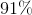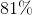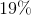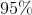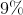Explanation:

If the correlation coefficient is 0.3, then the proportion of variance explained by the independent variable is 9 percent.

In other words, the correlation coefficient is denoted as follows: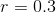The explained variance is found using the following formula: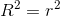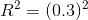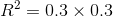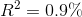Thus, 81% of the variance remains unexplained by variations in the independent variable.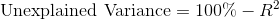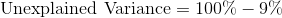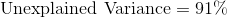Example Question #1 : Cognition

Which of the following lobes of the brain is involved in executive functions such as self-control, planning, reasoning, and abstract thought?

Occipital lobe

Temporal lobe

Parietal lobe

Frontal lobe